##### PostgreSQL CURRENT_TIME Function With Practical Examples

Postgres offers numerous date/time functions such as CURRENT_TIMESTAMP, CURRENT_DATE, NOW(), etc. The CURRENT_TIME is one of the date/time functions that retrieve the current time and the time zone value. This blog post will teach you the basic syntax, usage, and practical implementation of Postgres’ CURRENT_TIME function.

How to Use the CURRENT_TIME Function in PostgreSQL?

To use the CURRENT_TIME function in Postgres, the below-mentioned syntax must be followed:

`CURRENT_TIME(prec);`

The CURRENT_TIME function may accept an optional parameter “precision” that is used to set the precision of the retrieved fractional seconds. If the precision parameter is omitted, the fractional seconds will be retrieved with the full precision available. The return type of the CURRENT_TIME is TIMETZ.

Let’s go through the below examples to perceive the working of the CURRENT_TIME function.

Example 1: What Does Postgres' CURRENT_TIME Function Do?

Executing the below statement will retrieve the current time and timezone:

`SELECT CURRENT_TIME;`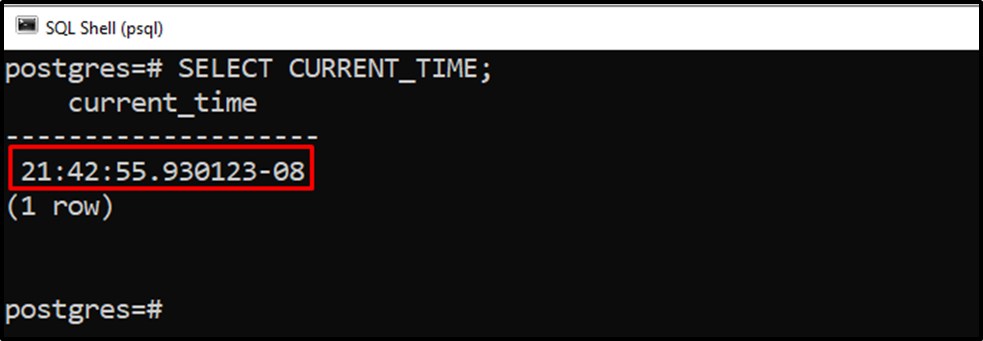The output snippet shows that the CURRENT_TIME function retrieves the current time and zone.

Example 2: How Does the CURRENT_TIME Function Work With Precision Parameter in Postgres?

Let’s learn how to use the CURRENT_TIME function with the precision parameter:

`SELECT CURRENT_TIME(3);`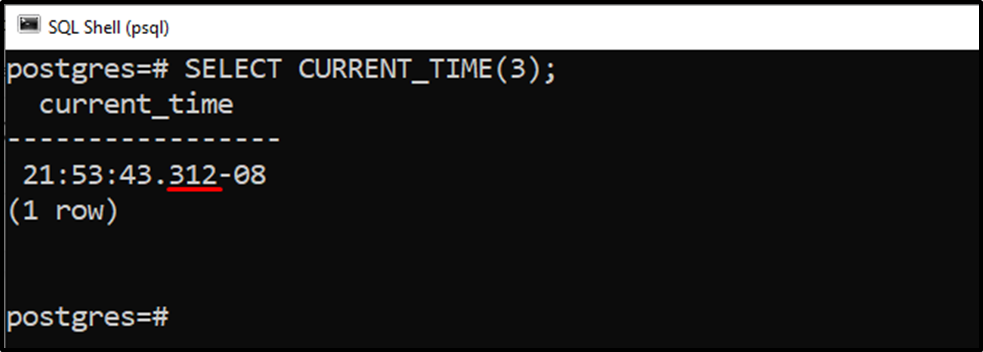From the output snippet, it is clear that the CURRENT_TIME function retrieves the current time based on the specified precision parameter.

Example 3: How to Use the CURRENT_TIME Function as the Column’s Default Value?

Let’s create a sample table named “students_login” with three columns “student_id”, “student_name”, and “student_login”:

```CREATE TABLE students_login(
student_id SERIAL PRIMARY KEY,
student_name TEXT,
);```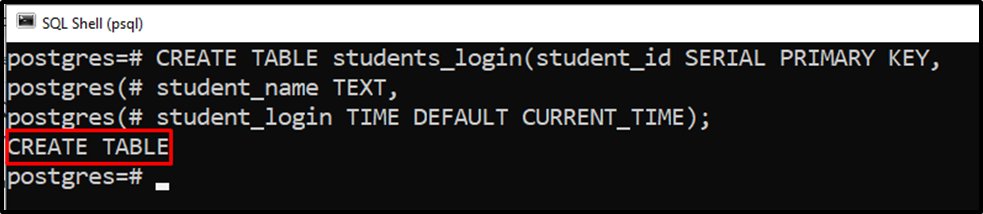In the above code, we utilize the DEFAULT keyword to assign the current time as a default time to the student_login column:

The “students_login” table has been created successfully. Let’s insert the student details via the “INSERT INTO” command:

```INSERT INTO students_login (student_name)
VALUES ('Joseph');```

In the above snippet, we specified only the student’s name; the current time will be assigned to the student_login column by default: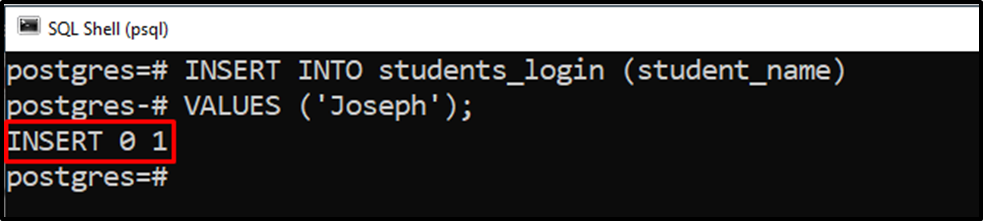Now, run the “SELECT *” command to check if a record has been inserted into the students_login table or not:

`SELECT * FROM students_login;`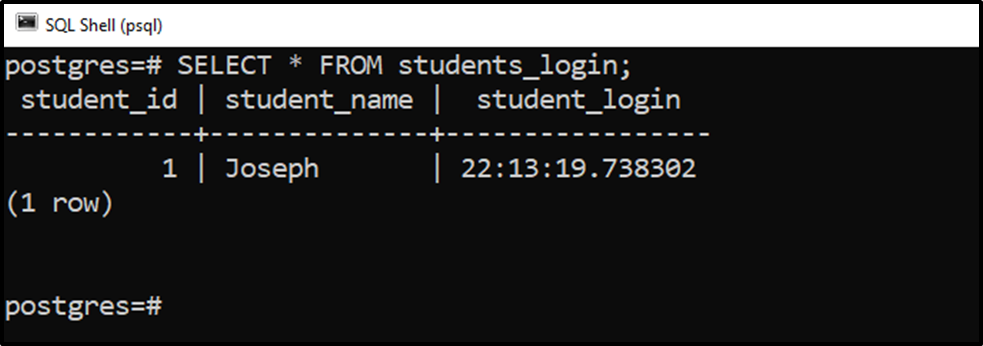The output snippet indicates that a student named “Joseph”, having a student id “1”, logged in at “22:13:19.738302”.

Let’s insert one more record to understand the working of the CURRENT_TIME function more clearly:

```INSERT INTO students_login (student_name)
VALUES ('Stephen');```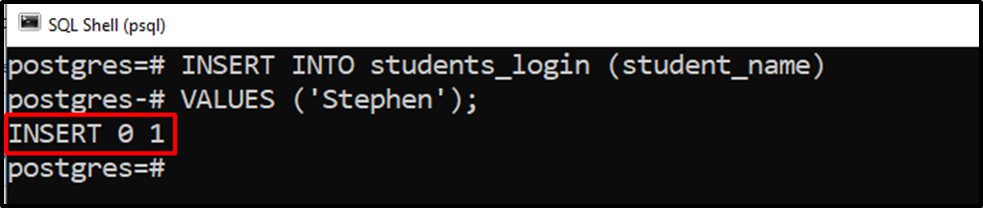The output snippet verifies that one more record has been inserted into the students_login table. To verify the details regarding the newly inserted record, run the “SELECT *” command:

`SELECT * FROM students_login;`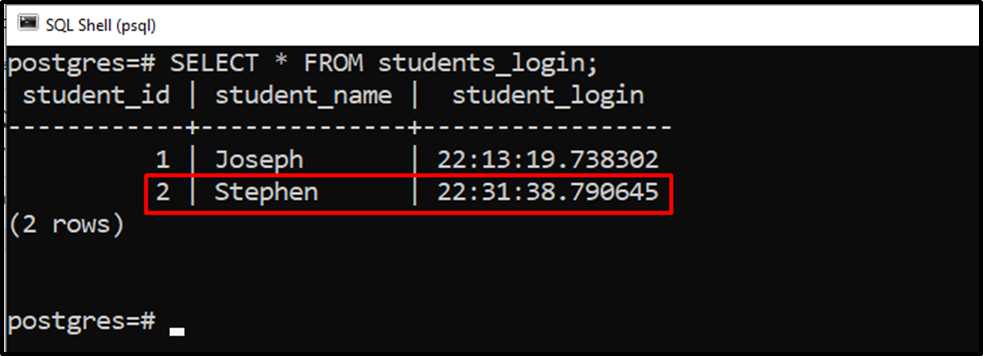The output snippet shows that another student named “Stephen”, having a student id “2”, logged in at “22:31:38.790645”.

This is how the CURRENT_TIME function works in PostgreSQL.

Conclusion

The CURRENT_TIME function is one of the date/time functions that retrieve the current time and the time zone. The CURRENT_TIME function may accept an optional parameter “precision” to set the precision of the retrieved fractional seconds. If the precision parameter is omitted, the fractional seconds will be retrieved with the full precision available. This Postgres blog demonstrated the working of the CURRENT_TIME function with various examples.